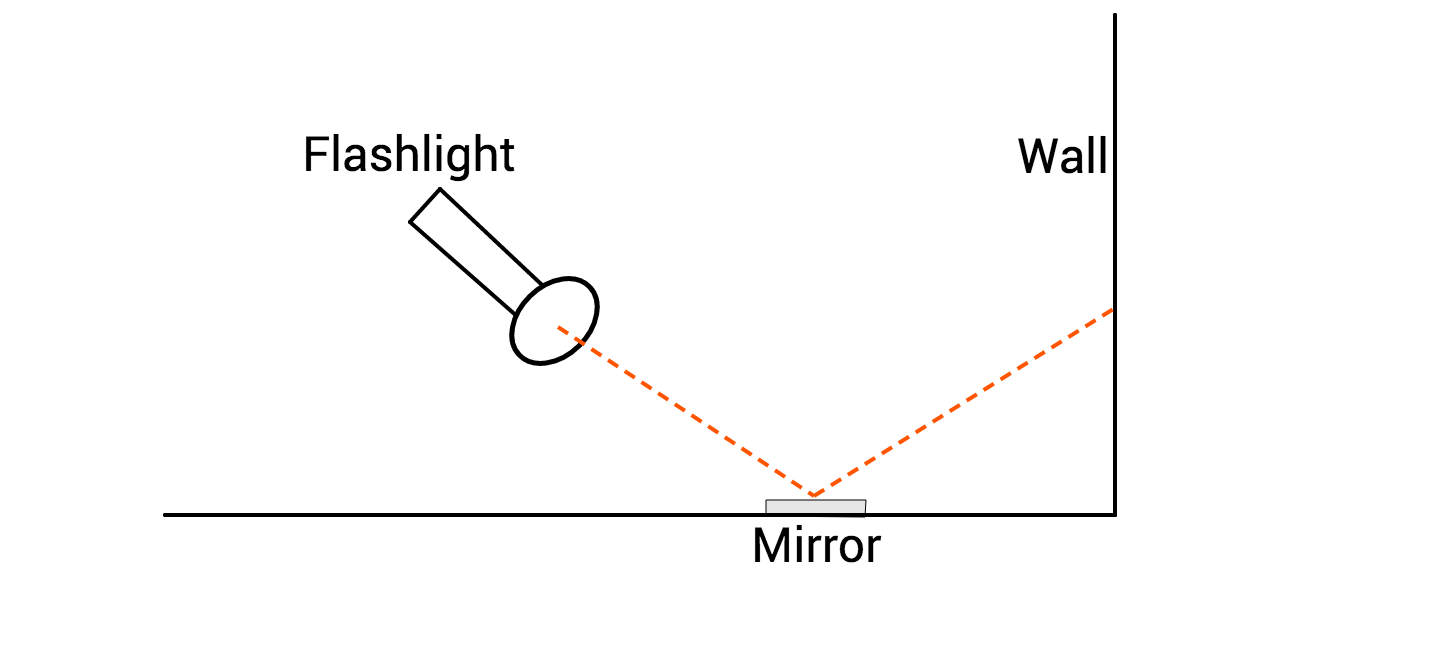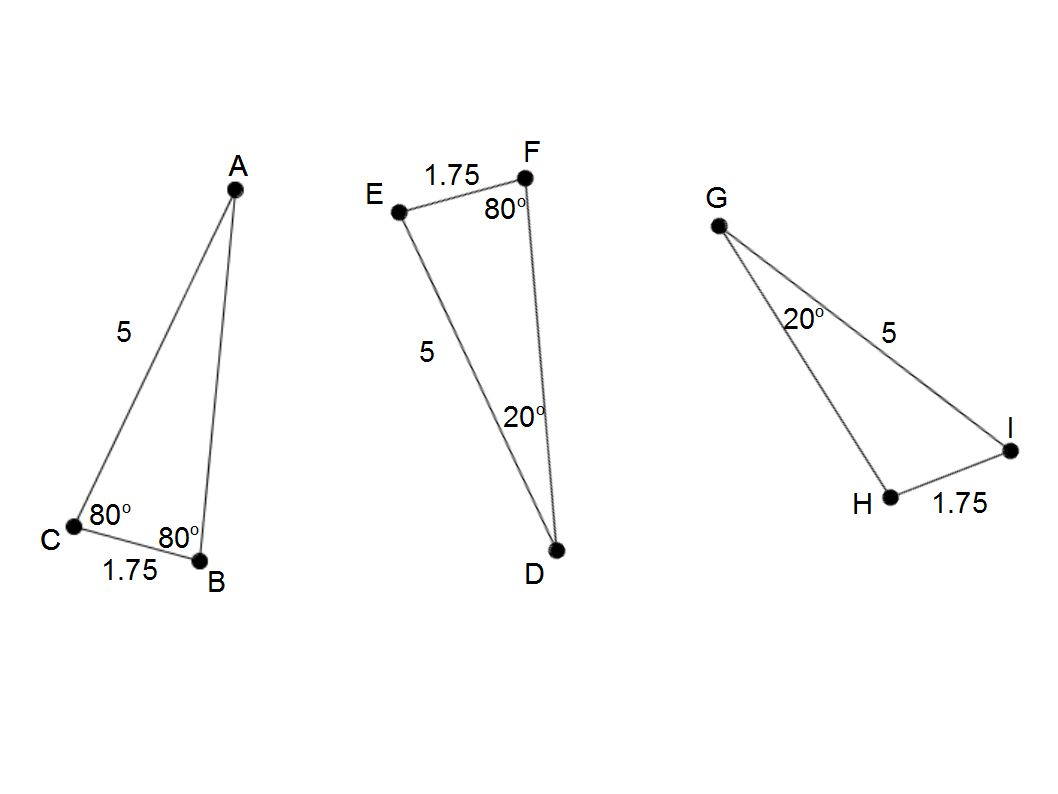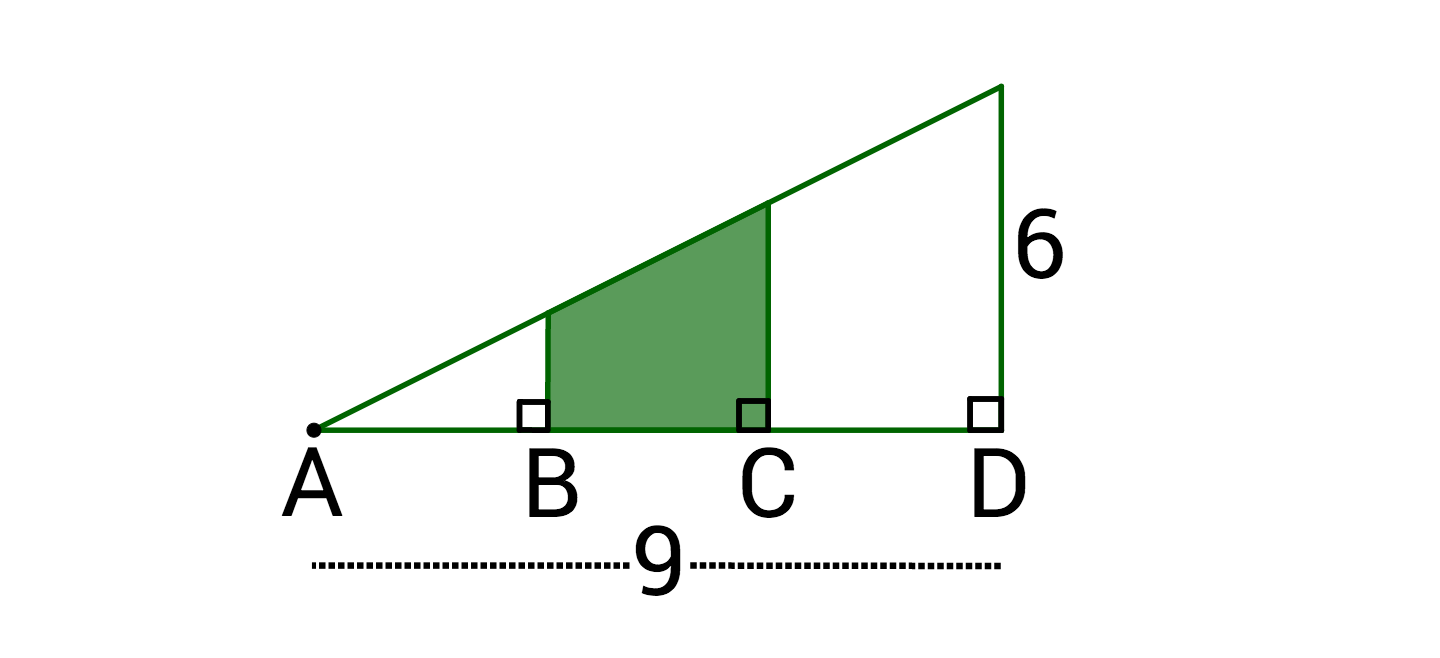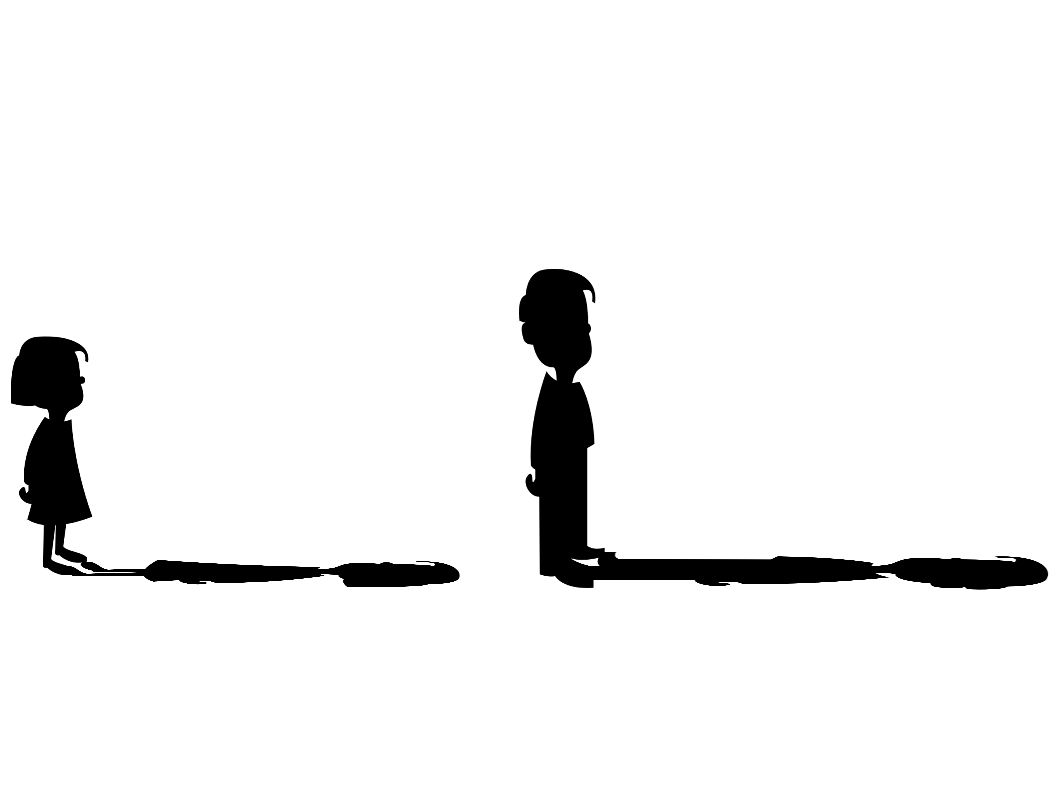Geometry

Similar and Congruent Triangles WarmupStephanie shines her flashlight into a mirror on the floor and sees the ray of light hit the wall on the other side of the room. As she moves closer to the mirror, holding the light at the same height, how will the ray of light on the wall change?

Which of the following triangles is not necessarily congruent to the other two?If $AB = BC = CD,$ what is the area of the green region?

Two triangles have the same angle measures of $60^\circ, 80^\circ, \text{ and } 40^\circ.$ Are the triangles congruent?

On a sunny day, Betty's shadow is 12 feet long and she is 4 feet tall. If Adam's shadow is 3 feet longer than Betty's, how tall is Adam?×